# Energy Profiles (Energy Diagrams) Chemistry Tutorial

## Key Concepts

⚛ An Energy Profile is also referred to as an Energy Diagram or as a Potential Energy Diagram.(1)

⚛ An energy profile is a diagram representing the energy changes that take place during a chemical reaction.

Enthalpy change, ΔH, is the amount of energy absorbed or released by a chemical reaction.(2)

On an energy profile, the enthalpy change for the reaction is measured from the energy of the reactants to the energy of the products.

Exothermic reaction, energy is released by the reaction.

On an energy profile, the energy of the reactants is greater than the energy of the products.

Endothermic reaction, energy is absorbed by the reaction.

On an energy profile, the energy of the reactants is lower than the energy of the products.

⚛ Activation Energy of a reaction, Ea or EA, is the minimum amount of energy reactant molecules must possess in order to form products.(3)

On an Energy Profile, the activation energy is measured from the energy of the reactants to the peak of the energy profile diagram.
The lower the activation energy, the faster the reaction will proceed.

⚛ Catalysts speed up the rate of reaction by lowering the activation energy without being consumed by the reaction.

A catalyst does not alter the enthalpy change value for the reaction.

⚛ An enzyme is a biological catalyst.

It contains active sites on its surface. These sites are capable of bonding only to specific molecules called substrates.
The lock and key theory proposes that the active site is a cavity of fixed shape which only a substrate molecule can fit into properly to undergo reaction.

⚛ Inhibitors (negative catalysts) are substances which slow down, or inhibit, a reaction.

Surface catalysed reactions can be inhibited when a foreign substance bonds at the catalyst's active sites blocking them for substrate molecules.
This type of inhibition is called poisoning and the inhibitor (negative catalyst) is called a poison.

No ads = no money for us = no free stuff for you!

## Energy Profile for Exothermic Reactions

The synthesis of ammonia gas (NH3(g)) from nitrogen gas (N2(g)) and hydrogen gas (H2(g)) is an exothermic reaction.
92.4 kJ mol-1 (of N2(g)) is released.
Energy (heat) is a product of the reaction:

N2(g) + 3H2(g) → 2NH3(g) + 92.4 kJ mol-1

In order for energy to be conserved during the chemical reaction, the "energy of the reactants" must be greater than the "energy of the products".

energy of reactants = energy of products + energy released

energy of N2(g) and H2(g) = energy of NH3(g) + 92.4 kJ mol-1

Chemists refer to the "energy of the reactants" as their enthalpy, Hreactants.
Enthalpy of products, Hproducts, is the "energy of the products".

So, we can write:

enthalpy of reactants = enthalpy of products + energy released

H(N2(g) and H2(g)) = H(NH3(g)) + 92.4 kJ mol-1

We know the enthalpy change for the reaction: ΔH = -92.4 kJ mol-1.
(Remember the minus sign (-) tells us energy is released, energy is a product of the reaction, the reaction is exothermic.)
If we assume the total enthalpy of the reactants is 192.4 kJ mol-1, then we calculate the enthalpy of the products:
enthalpy of products = enthalpy of reactants - 92.4 = 192.4 - 92.4 = 100 kJ mol-1.
We could sketch a diagram to show the relative enthalpies of reactants, H(N2(g) and H2(g)), and products, H(NH3(g)), and the enthalpy change for the reaction (ΔH), as shown below:

 energy (kJ mol -1) Relative Enthalpy of Reactants and Productsreaction coordinate → (reaction path →)

Note that the energy of the reactants is greater than the energy of the products by an amount equal to the energy that is released by the reaction (92.4 kJ mol-1).
The x-axis is labelled "reaction coordinate" or "reaction path". The reaction coordinate tells us about the energy of the system at a particular stage of the reaction.(4)
Initially at stage 1, or the first coordinate, only the energy of the reactant molecules is being considered.
On the diagram above the final stage, or the final coordinate, of the reaction is when the energy of product molecules are considered but not reactant molecules.
The reaction coordinate (reaction path) is not the same as time.

But, we have a problem.
The air we breathe is made up of about 78% nitrogen gas (N2(g)) and a tiny amount (about 0.00005%) of hydrogen gas (H2(g)), and, no measurable ammonia on this scale.
If N2(g) and H2(g) easily react to form NH3(g), there shouldn't be any hydrogen gas in the atmosphere but we should be detecting ammonia gas instead of hydrogen gas! And, everytime we make hydrogen gas, for example by reacting an active metal with hydrochloric acid, then that hydrogen gas should immediately react with the nitrogen gas in the air to produce ammonia, and we should detect ammonia (by its smell) and not hydrogen gas (by the "pop test").
There must be some "barrier" that prevents the nitrogen gas and hydrogren gas in the atmosphere reacting to form ammonia gas.
In order for reactants to react, they need to have a minimum amount of energy. If the reactant molecules have this minimum amount of energy, then, when the reactant molecules collide, they can react to form product molecules (which we call successful or fruitful collisions). If the reactant molecules do not have this minimum amount of energy, then collisions between reactant molecules will not be successful and product molecules will not be produced.
The amount of energy we need to supply in order for N2(g) and H2(g) molecules to collide successfully must be quite large, otherwise the nitrogen and hydrogen molecules in our atmosphere would successfully collide with each other to form ammonia gas in the atmosphere.
We can refer to this "extra energy" we need to supply as an "energy barrier".
Our energy diagram needs to be ammended to show the reactant molecules absorbing some energy before the product molecules can be made.

Let's assume the "energy barrier" is 100 kJ mol-1, that is, the reactant molecules must absorb 100 kJ mol-1 of energy before they have sufficient energy to allow for successful collisions between nitrogen molecules and hydrogen molecules.
Our sketch of the relative enthalpy of reactants and products needs to include a new stage, or coordinate, representing this absorbed energy.
The new diagram now looks like the one shown below:

 energy (kJ mol-1) Relative Enthalpy of Reactants and Productsreaction coordinate → (reaction path →)

Chemists call this "energy barrier" the "activation energy" for the chemical reaction.
Activation energy is usually given the symbol Ea.
Activation energy represents the minimum amount of energy that must be absorbed by the reactant molecules before they can collide successfully and produce product molecules.

Once reactant molecules have sufficient energy they collide and form a high-energy intermediate product known as the activated complex.
This activated complex is unstable, as soon as it forms it breaks apart into the molecules that make up the products of the reaction, releasing energy in the process.
This activated complex stage of the reaction must be very short.
Therefore our sketch of the relative energies of reactants and products for our reaction, needs to show the highest energy achieved as a point, not a line, on the energy diagram.
The ammended diagram, which we now refer to as an "energy profile" is shown below:

 energy (kJ mol-1) Energy Profilereaction coordinate → (reaction path →)

This energy profile shows us the:

(1) activation energy (Ea) required to allow the reaction to proceed in the forward direction is 100 kJ mol-1, Ea = 100 kJ mol-1
(reactant molecules must absorb 100 kJ mol-1 of energy before collisions will be successful)

(2) enthalpy change for the forward reaction, ΔH, is -92.4 kJ mol-1, ΔH = -92.4 kJ mol-1
Hreaction = Hproducts - Hreactants = -92.4 kJ mol-1)

## Energy Profile for Endothermic Reactions

We saw above that the synthesis of ammonia gas from nitrogen gas and hydrogen gas was an exothermic process:

N2(g) + 3H2(g) → 2NH3(g) + 92.4 kJ mol-1

and we constructed an Energy Profile to show the relative enthalpies of reactants and products.

Now consider the decomposition of ammonia gas (NH3(g)) to produce hydrogen gas (H2(g)) and nitrogen gas (N2(g)).
This reaction will be the reverse of the ammonia synthesis reaction above, that is, the chemical equation for the decomposition of ammonia gas is:

2NH3(g) + 92.4 kJ mol-1 → N2(g) + 3H2(g)

and the energy profile for the decomposition reaction will also be the "reverse" of that for the synthesis reaction:

 energy (kJ mol-1) Energy Profilereaction coordinate → (reaction path →)

Note that the reactant (NH3(g)) molecules must now absorb 92.4 + 100 = 192.4 kJ mol-1 of energy in order to give them sufficient energy for successful (or fruitful) collisions to occur resulting in product molecules.
The activation energy for this reaction is 192.4 kJ mol-1.
Ea = 192.4 kJ mol-1

Once the reactant molecules have absorbed this amount of energy (the activation energy, Ea), the high-energy intermediate product known as the activated complex will form.
As soon as the activated complex forms, it breaks apart, releasing energy and forming the products of the reaction.
From our energy profile diagram we see that 192.4 kJ mol-1 of energy was absorbed by the reactant molecules, but only 100 kJ mol-1 was released as the activated complex broke apart to make the product molecules.
Overall, the system absorbed a net amount of energy of 192.4 - 100 = 92.4 kJ mol-1.
In other words, the difference in the enthalpy of the products and reactants is 92.4 kJ mol-1.
Because the reaction is endothermic, energy is absorbed by the system, the value for the enthalpy change, ΔH, is positive (+), ΔH = +92.4 kJ mol-1.

## Effect of a Catalyst on an Energy Profile

A catalyst can be used to increase the rate of a reaction.
A catalyst DOES NOT change:

⚛ the enthalpy of the reactants (Hreactants)

⚛ the enthalpy of the products (Hproducts)

⚛ the enthalpy change for the reaction (ΔH)
since ΔH = Hproducts - Hreactants

A catalyst DOES lower the activation energy required for the reaction to proceed.
The catalyst provides an alternative, lower-energy, pathway for the reaction to follow, using a lower-energy intermediate product (lower-energy activated complex).
If the catalyst is a solid, it can do this by providing a surface on which the reactant molecules can "stick" in the correct orientation, increasing the rate at which successful collisions occur.

A number of solid catalysts are available for increasing the rate of commercial ammonia gas production (see the Haber Process tutorial).
mLet's consider a catalyst that is capable of reducing the activation energy for the synthesis of ammonia gas by 50%.
That is, instead of requiring an activation energy of 100 kJ mol-1, the activation energy for the reaction is decreased to just 50 kJ mol-1.
The energy profile for the reaction would now look like the one below:

 energy (kJ mol-1) Energy Profilereaction coordinate → (reaction path →)

Note that the catalyst lowers the activation energy for both the forward and reverse reactions.

For the forward reaction,

N2(g) + 3H2(g) → 2NH3(g)     ΔH = -92.4 kJ mol-1
Ea(catalysed) = 50 kJ mol-1
Ea(uncatalysed) = 100 kJ mol-1

For the reverse reaction,

2NH3(g) → N2(g) + 3H2(g)     ΔH = +92.4 kJ mol-1
Ea(catalysed) = 50 + 92.4 = 142.4 kJ mol-1
Ea(uncatalysed) = 100 + 92.4 = 192.4 kJ mol-1

So, the rate of the forward reaction will increase for the catalysed reaction, and, the rate of the reverse reaction will also increase for the catalysed reaction.

Note that you could find a substance that slows down the rate of the forward and reverse reactions by increasing the activation energy for the reaction.
This kind of substance has the opposite effect to a catalyst, so it is sometimes known as a negative catalyst, but is more often known as an inhibitor because it inhibits the reaction.

## Summary of Energy Profiles (Energy Diagrams)

The table below provides a summary of the energy profiles (energy diagrams) for fast and slow exothermic and endothermic reactions with or without the use of a catalyst:

Type of Reaction Faster Reaction
Lower activation energy
Slower Reaction
Higher activation energy
Effect of a Catalyst
A catalyst lowers the activation energy
Exothermic
Reaction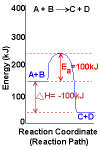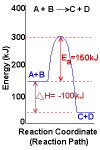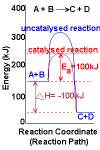Endothermic
Reaction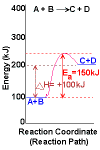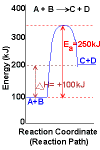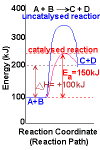## Problem Solving: Energy Profiles (Energy Diagrams)

Below is a worked example of finding the enthalpy change for a reaction given the Energy Profile using the StoPGoPS approach to problem solving in chemistry.

The Problem: The energy profile below is for the decomposition of N2O4(g) into NO2(g).
 energy (kJ mol-1) Energy Profilereaction coordinate → (reaction path →)

Determine the enthalpy change for the reaction:

N2O4(g) → 2NO2(g)

Solving the Problem

 STOP! State the question. What is the question asking you to do? Determine (calculate) the enthalpy change for the reaction ΔH = ? kJ mol-1 PAUSE! Pause to Plan. What information (data) have you been given? Read off the energy profile: enthalpy of reactants: H(N2O4(g)) = 250 kJ mol-1 enthalpy of products: H(2NO2(g)) = 50 kJ mol-1 What is your plan for solving this problem? The relationship between the enthalpy change for the reaction and the enthlpy of the reactants and products is given by the equation: ΔH(reaction) = H(products) - H(reactants) ΔH(reaction) = H(2NO2(g)) - H(N2O4(g)) GO! Go with the Plan. Substitute the data into the equation to calculate the enthalpy change ΔH(reaction) = H(2NO2(g)) - H(N2O4(g)) ΔH(reaction) = 50 - 250 = -200 kJ mol-1 PAUSE! Ponder Plausability. Have you answered the question that was asked? Yes, we have calculated the enthalpy for the reaction in kJ mol-1 Is your solution to the question reasonable? The energy profile clearly shows that the energy of the products is much lower than the energy of the reactants: reactants → energy + products Therefore the reaction releases energy, it is exothermic, so the enthalpy change for the reaction (ΔH) must be negative. We can work backwards, using the value for the enthalpy of reactants (250 kJ mol-1) and the enthalpy change for the reaction (-200 kJ mol-1) to calculate the enthalpy of the products: ΔH = H(products) - H(reactants) -200 = H(products) - 250 -200 + 250 = H(products) +50 = H(products) Since this value for H(products) agrees with what we can read off the energy profile, we are reasonably confident that our value for ΔH is plausible. STOP! State the solution. What is the enthalpy change for the reaction? ΔH = −200 kJ mol-1

## Sample Question: Energy Profiles

The energy profile shown below represents the energy changes over the course of the following chemical reaction:

CO(g) + NO2(g) → CO2(g) + NO(g)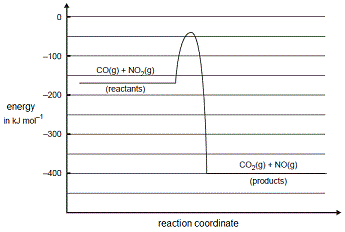Determine the enthalpy change for the reverse reaction.

ΔH = kJ mol-1

Footnotes:

(1) Another term you may see used is Gibbs energy diagram.

(2) Enthalpy refers to the internal energy of the system plus the product of pressure and volume. Enthalpy change in a system is equal to the heat brought to the system at constant pressure.

(3) Symbols used in this tutorial are those recommended in the IUPAC Green Book "Quantities, Units and Symbols in Physical Chemistry" Third Edition

(4) The reaction coordinate is actually a measureable geometric parameter that changes during the conversion of reactant species into product species.
For example, a bond length and/or bond angle could be monitored during the course of a chemical reaction.